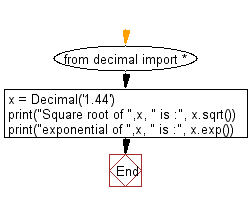﻿ Python Math: Square root and exponential of the specified decimal number - w3resource# Python Math: Square root and exponential of a given decimal number

## Python Math: Exercise-38 with Solution

Write a Python program to get the square root and exponential of a given decimal number.

Sample Solution:-

Python Code:

``````from decimal import *
x = Decimal('1.44')
print("Square root of ",x, " is :", x.sqrt())
print("exponential of ",x, " is :", x.exp())
```
```

Sample Output:

```Square root of  1.44  is : 1.2
exponential of  1.44  is : 4.220695816996552825673328929
```

Flowchart:Python Code Editor:

Have another way to solve this solution? Contribute your code (and comments) through Disqus.

What is the difficulty level of this exercise?

Test your Programming skills with w3resource's quiz.

﻿

```>>> students = [{'name': 'John', 'score': 98}, {'name': 'Mike', 'score': 94}, {'name': 'Jennifer', 'score': 99}]